You are currently offline. Some features of the site may not work correctly.

# Menger's theorem

Known as: Menger's n-arc theorem, Menger, Erdős–Menger conjecture
In the mathematical discipline of graph theory and related areas, Menger's theorem is a characterization of the connectivity in finite undirected… Expand
Wikipedia

## Papers overview

Semantic Scholar uses AI to extract papers important to this topic.
2013
2013
• Inf. Sci.
• 2013
• Corpus ID: 8470528
The concept of the strongest path plays a crucial role in fuzzy graph theory. In classical graph theory, all paths in a graph are… Expand
2005
2005
• 2005
• Corpus ID: 17403164
Abstract.We prove that the Hubbard model at finite temperature T and half-filling is analytic in its coupling constant λ for… Expand
•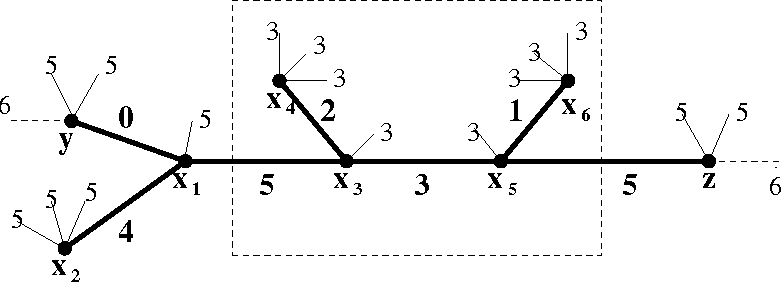•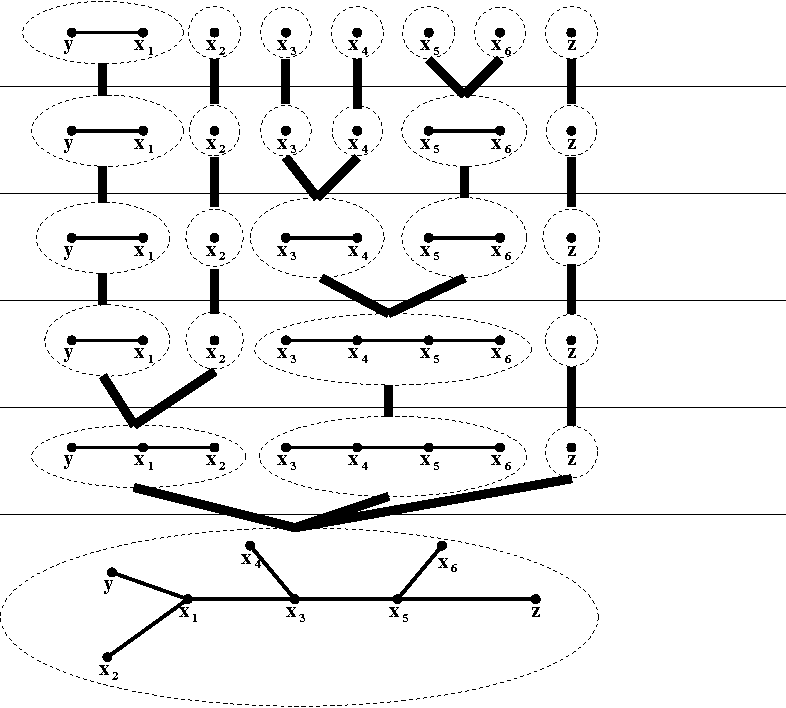•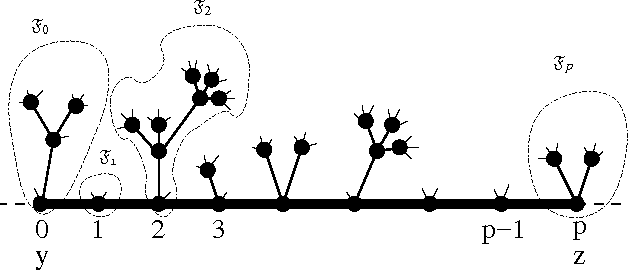•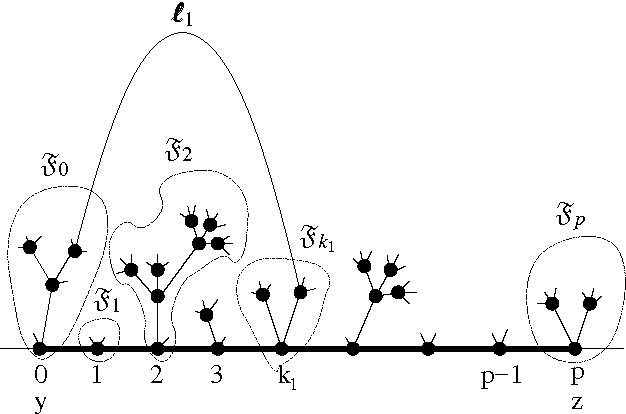•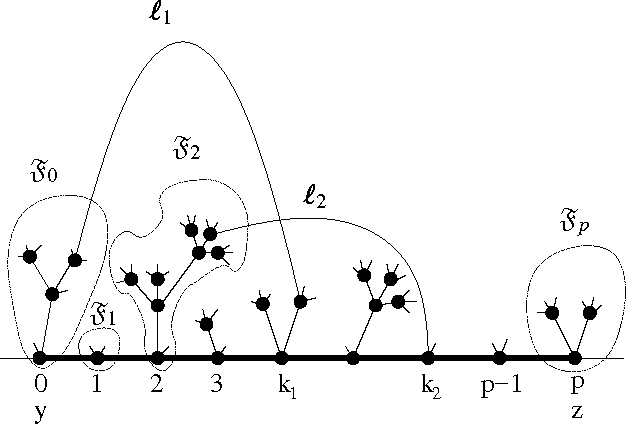2005
2005
• Theor. Comput. Sci.
• 2005
• Corpus ID: 34838595
In the minimun path coloring problem, we are given a graph and a set of pairs of vertices of the graph and we are asked to… Expand
2001
2001
• J. Graph Theory
• 2001
• Corpus ID: 9370918
Menger's Theorem for digraphs states that for any two vertex sets A and B of a digraph D such that A cannot be separated from B… Expand
2001
2001
• 2001
• Corpus ID: 121779860
Menger's Theorem for digraphs states that for any two vertex sets A and B of a digraph D such that A cannot be separated from B… Expand
Highly Cited
1996
Highly Cited
1996
• Comb.
• 1996
• Corpus ID: 206792815
AbstractA graph with at least 2k vertices is said to bek-linked if, for any choices1,...,sk,t1,...,tkof 2k distinct vertices… Expand
1991
1991
• Comb.
• 1991
• Corpus ID: 206793996
An(a, b)-n-fan means a union ofn internally disjoint paths. Menger's theorem states that a graphG has an(a, b)-n-fan if and only… Expand
1987
1987
• R. Aharoni
• J. Comb. Theory, Ser. B
• 1987
• Corpus ID: 17907913
Abstract The countable case of a conjecture of Erdos is settled: let G = (V, E) be a directed or undirected graph, where V is… Expand
1984
1984
A proof of Menger’s theorem is presented. We use the notation and terminology of Bondy and Murty [ll. Let D be a directed graph… Expand
1983
1983
Abstract Menger's theorem can be stated as follows: Let G = ( V , E ) be a finite graph, and let A and B be subsets of V . Then… Expand